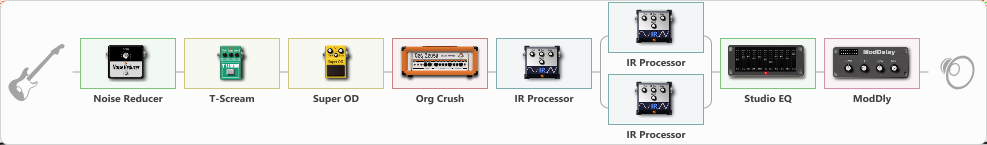# Randall sound

Discussion in 'ToneLib-GFX presets' started by Tony the Metalhead, Dec 6, 2021.

1. ### Tony the MetalheadActive Member

Randall sound

Preset name: Orange Randall

Effects chain:Effect: "Noise Reducer" (Dynamics / Filter), active - "yes"
{
"Sens" = 77
"Mode" = Hard
}

Effect: "T-Scream" (Overdrive / Distortion), active - "yes"
{
"Drive" = 20
"Tone" = 40
"Level" = 10
}

Effect: "Super OD" (Overdrive / Distortion), active - "yes"
{
"Drive" = 60
"Tone" = 60
"Level" = 40
}

Effect: "Org Crush" (Amp simulators), active - "yes"
{
"Gain" = 100
"Bass" = 20
"Middle" = 5
"Treble" = 65
"Presence" = 65
"Master" = 30
"Level (dB)" = 6
}

Effect: "IR Processor" (Cabinets), active - "yes"
{
"IR" = Randall667
"Low Cut (Hz)" = 125
"Hi Cut (kHz)" = 20.0
"Mix" = 100
"Level (dB)" = 6
}

Effect: "Splitter" (Dynamics / Filter)
{
"A-Bypass" = Off
"A-Pan" = 0
"A-Level" = 50
"B-Bypass" = Off
"B-Pan" = 0
"B-Level" = 55

'A' branch:
{

Effect: "IR Processor" (Cabinets), active - "yes"
{
"IR" = OD-UK112-V30-DYN-57-P10-20
"Low Cut (Hz)" = 100
"Hi Cut (kHz)" = 20.0
"Mix" = 100
"Level (dB)" = -2
}
}
'B' branch:
{

Effect: "IR Processor" (Cabinets), active - "yes"
{
"IR" = OD-UK112-V30-DYN-57-P09-40
"Low Cut (Hz)" = 80
"Hi Cut (kHz)" = 20.0
"Mix" = 100
"Level (dB)" = 0
}
}
}

Effect: "Studio EQ" (Dynamics / Filter), active - "yes"
{
"31 Hz" = -2
"62 Hz" = -3
"125 Hz" = -4
"250 Hz" = -7
"500 Hz" = -8
"1 kHz" = -8
"2 kHz" = -8
"4 kHz" = -8
"8 kHz" = -9
"16 kHz" = 10
"Level (dB)" = 0
}

Effect: "ModDly" (Delay), active - "yes"
{
"Time" = 282
"Feedback" = 25
"Tone" = 35
"Speed" = 1.1
"Mix" = 25
}

Note: You will need to download and install the ToneLib-GFX software to use the preset.

#### Attached Files:

• ###### Randall_sound.tlgfx
File size:
7.6 KB
Views:
2,161
tonyyyyy and Buggy Ramirez like this.